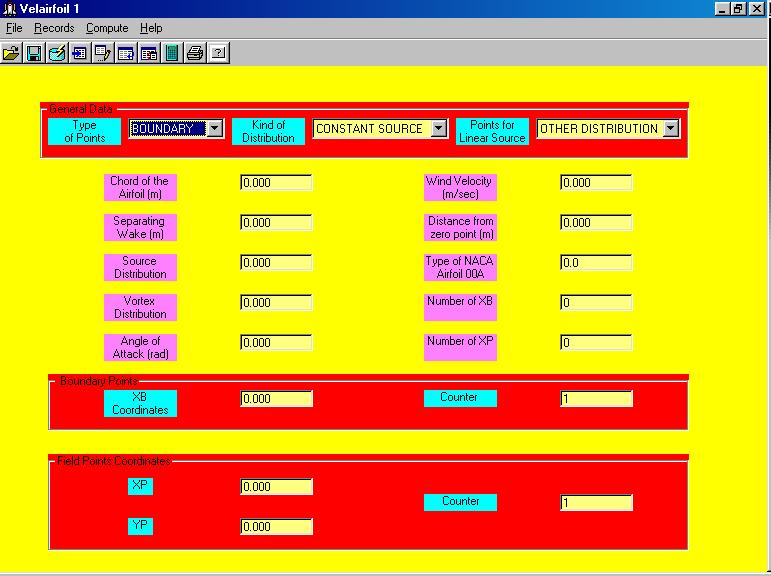Program 1 : Two-dimensional Aerodynamics (airfoil without velocity) (Velairfoil 1)A. GENERAL INFORMATION

1. The current program is a result of a general non - linear model used for the determination of the velocity and pressure coefficient field around a NACA airfoil in two - dimensional unsteady inviscid flow.

2. This nonlinearity resulted, because of the general form given to the source and vortex strength distribution, while these are dependent on the history of the vorticity and source distribution on the NACA airfoil surface.

3. For the special cases where the source and vortex strength distribution are either linear or constant, the general non - linear problem is reduced to a simpler linear.

The following linear cases are covered by the program:

A. Linear Vortex Distribution.

B. Linear Source Distribution.

C. Constant Vortex Distribution.

D. Constant Source Distribution.

B. SPECIAL INFORMATION

1. Separating wake should be greater than two times chord of the airfoil.

2. For the cases of Linear Source or Linear Vortex Distribution, angle of attack should be different than zero, and vortex and source distribution equal to zero.

3. For the case of Constant Source Distribution, the vortex distribution should be zero.

4. For the case of Unit Source Distribution, the source distribution should be one.

5. For the case of Constant Vortex Distribution, the source distribution should be zero.

6. For the case of Unit Vortex Distribution, the vortex distribution should be one.

C. DATA ENTRY - OUTPUT RESULTS

1. The program opens a file *.dat (data entry). This file includes all the requested data.

2. The results of the program are given by a file *.res (output results).

3. Both data entry (file *.dat) and output results (file *.res) are printed by the program.

D. KEYS OF THE PROGRAM

The Records are referred either to Boundary Points Coordinates or Field Points Coordinates.

1. Add Records: Records are added by placing in Counter a number 1,2,3, ...etc.and then by pressing key "Add".

2. Edit Records: Records are edited by placing in Counter a number 1,2,3, ...etc and then by pressing key "Edit".

3. Erase Records: In order to erase a specific Coordinate, write in Counter its corresponding number and then press "Erase".

4. Insert Records: In order to insert a specific Coordinate between two other existing coordinates, write in Counter its corresponding number and then press "Insert".